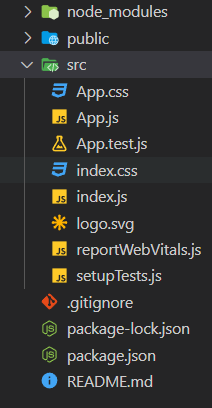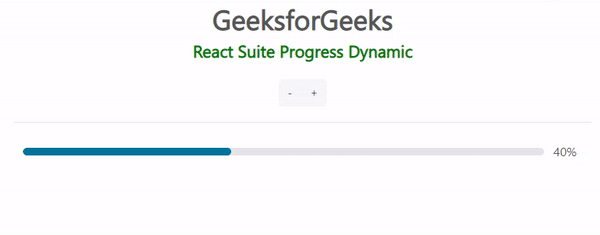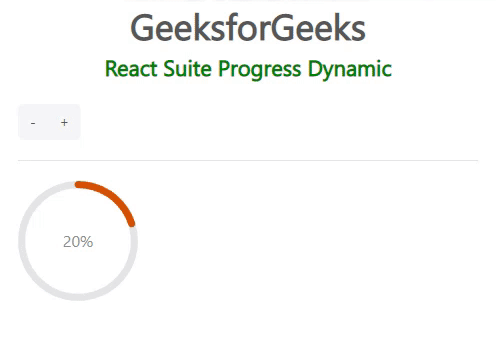GFG App
Open AppBrowser
Continue

React suite is a library of React components, sensible UI design, and a friendly development experience. It is supported in all major browsers. It provides pre-built components of React which can be used easily in any web application. In this article, we’ll learn about React suite Progress Dynamic.

The progress component allows the user to display the current progress of an operation flow. Dynamic progress can be created in which the progress can be displayed dynamically.

Progress.Line Props:

• classPrefix: It is used to indicate the component CSS class’s prefix.
• percent: It is used to set the completion percentage.
• showInfo: It is used to indicate whether to display text or not.
• status: It is used to set the status of the Progress.
• strokeColor: It is used to denote the line color.
• strokeWidth: It is used to set the line width.
• vertical: The progress bar is vertically displayed.

Progress.Circle Props:

• classPrefix: It is used to denote the prefix of the component CSS class
• gapDegree: It is used to denote the gap degree of the half-circle.
• gapPosition: It is used to denote the gap position.
• percent: It is used to set the completion percentage.
• showInfo: It is used to indicate whether to display text or not.
• status: It is used to set the status of the Progress.
• strokeColor: It is used to denote the line color.
• strokeLinecap: It is used to denote the end of different types of open paths
• strokeWidth: It is used to set the line width.
• trailColor: It is used to set the unfilled part color.
• trailWidth: It is used to set the unfilled part width.

Syntax:

```const [
percent,
setPercent
] = useState(...);

const decline = () => {
...
}
const increase = () => {
...
}
function App() {
<Progress.Line
percent={percent}
strokeColor={color}
/>
}```

Creating React Application And Installing Module:

Step 1: Create a React application using the given command:

`npm create-react-app projectname`

Step 2: After creating your project, move to it using the given command:

`cd projectname`

Step 3: Now Install the rsuite node package using the given command:

`npm install rsuite`

Project Structure: Now your project structure should look like the following:Example 1: Below is the example code that demonstrates the Dynamic Progress Bar.

## Javascript

 `import ``"rsuite/dist/rsuite.min.css"``; ` `import { ` `    ``Progress, ` `    ``ButtonGroup, ` `    ``Button ` `} from ``"rsuite"``; ` `import { useState } from ``"react"``; ` ` `  `export ``default` `function` `App() { ` `    ``const [ ` `        ``percent, ` `        ``setPercent ` `    ``] = useState(40); ` ` `  `    ``const decrease = () => { ` `        ``const value = ` `            ``Math.max(percent - 10, 0); ` `        ``setPercent(value); ` `    ``}; ` ` `  `    ``const increase = () => { ` `        ``const value = ` `            ``Math.min(percent + 10, 100); ` `        ``setPercent(value); ` `    ``}; ` ` `  `    ``const status = ` `        ``percent === 100 ? ``"success"` `: ``null``; ` `    ``const color = ` `        ``percent === 100 ? ``"#03D613"` `: ``"#02749C"``; ` ` `  `    ``return` `( ` `        ``
` `            ``
` `                ``

GeeksforGeeks

` `                ``

React Suite Progress Dynamic ` `                ``

` `            ``
` `            ``
` `                ``
` `                    `` ` `                        `` ` `                        `` ` `                    `` ` `                    ``
` `                    `` ` `                ``
` `            ``
` `        ``
` `    ``); ` `}`

Output:Example 2: Below is another example that demonstrates the Dynamic Progress in a circle shape.

## Javascript

 `import ``"rsuite/dist/rsuite.min.css"``; ` `import { ` `    ``Progress, ` `    ``ButtonGroup, ` `    ``Button ` `} from ``"rsuite"``; ` `import { useState } from ``"react"``; ` ` `  `export ``default` `function` `App() { ` `    ``const [ ` `        ``percent, ` `        ``setPercent ` `    ``] = useState(20); ` ` `  `    ``const decrease = () => { ` `        ``const value = ` `            ``Math.max(percent - 10, 0); ` `        ``setPercent(value); ` `    ``}; ` ` `  `    ``const increase = () => { ` `        ``const value = ` `            ``Math.min(percent + 10, 100); ` `        ``setPercent(value); ` `    ``}; ` ` `  `    ``const status = ` `        ``percent === 100 ? ``"success"` `: ``null``; ` `    ``const color = ` `        ``percent === 100 ? ``"#03D613"` `: ``"#D65003"``; ` ` `  `    ``return` `( ` `        ``
` `            ``
` `                ``

GeeksforGeeks

` `                ``

` `                    ``React Suite Progress Dynamic ` `                ``

` `            ``
` `            ``
` `                ``
` `                    `` ` `                        `` ` `                        `` ` `                    `` ` `                    ``
` `                    ``
` `                        `` ` `                    ``
` `                ``
` `            ``
` `        ``
` `    ``); ` `}`

Output:My Personal Notes arrow_drop_up